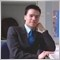• Overview
• Reviews (1)

Big MA15

BigMA15 is a fully automated EA. It is based on mathematical algorithms of the Stochastic Oscillator, ADX indicator and two moving averages, one short and another long. BigMA15 works by strategy based on above mentioned indicators.

EA is designed for EUR/USD, and it is optimized for M15 charts, although works with M5 charts.

Global Variables

• MAGIC= Number for the EAs orders.
• StopLoss= Number of pips to place the Stop Loss.
• TakeProfit= Number of pips to place the Take Profit.
• TradePct= Percent of the free margin to open a position. It is show as a percent division; 5%= 5/100 = 0.05 , TradePct=0.05
• MovingPeriodS= Period of the moving average short.
• MovingPeriodL= Period of the moving average long.
• ADX_Period= Period of the ADX indicator.
• K_Period= Period of K parameter, on Stochastic oscillator.
• D_Period= Period of D parameter, on Stochastic oscillator.
• S_Period= Period of S parameter, on Stochastic oscillator.
• ADX_Open= Value of main line of ADX indicator to open a position.
• TlP= Diference beetwen price current and price stop for can be modify a position.
• TsP= Number of pips to moving the stop loss when a position are modified.
• TpP= Number of pips to moving the take profit when a position are modified.
• MinHour= Hour to start trading.
• MaxHour= Hour to stop trading.
• MonHour= Hour to start trading on monday.
• FriHour= Hour to stop trading on friday.12660
2018.05.10 14:43

User didn't leave any comment to the rating